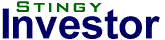Contact - Subscribe - LoginHome | Articles | Screens | Links | SNW | Rothery Report

The Stingy News Weekly (07/16/2018)

 "I quickly convinced myself that the true key to happiness lay in a modest standard of living which could be achieved with little difficulty under almost all economic conditions." - Ben Graham

Simple momentum
"This simple momentum strategy would have grown at 10.5% for the last 91 years, compared to 10.1% for the index."
[Momentum Investing]

Lessons from Benjamin Graham
"In June 2003, HarperCollins Publishers asked if I would be interested in updating Benjamin Graham's classic The Intelligent Investor. After determining that the proposal was not somebody's idea of a practical joke, I agreed to take on the project. And as I worked on revising the book, I was reminded, once again, what a genius Graham was."
[Graham]

Momentum's magic number
"We find similar results, with performance peaking when the formation period plus the holding period is equal to 12-to-14 months."
[Momentum Investing]

Aaron Brown on risk
"Aaron Brown talks about risk at market highs and lows." [video]
[Markets]

S&P/TSX60 Value Screens

 High Dividend Yield Stocks P/E P/B P/S Yield Inter Pipeline (IPL) C D E A Enbridge (ENB) E B B A BCE Inc. (BCE) C E C A Emera (EMA) E B B A Power Corp of Canada (POW) A A A A Pembina Pipeline (PPL) D C E A TransCanada (TRP) B D D A CIBC (CM) A B C A TELUS (T) C D C A Shaw (SJR.B) D D D A Notes | More Info...

 Low Price/Earnings Stocks P/E P/B P/S Yield Metro (MRU) A D A D Cenovus Energy (CVE) A A A D Teck Resources (TECK.B) A A B E Power Corp of Canada (POW) A A A A Magna (MG) A C A C CIBC (CM) A B C A Dollarama (DOL) A F B E Bank of Nova Scotia (BNS) A B C A National Bank (NA) A C C B Kinross Gold (K) A A B F Notes | More Info...

 Low Price/Book Value Stocks P/E P/B P/S Yield Crescent Point (CPG) F A B B Goldcorp (G) C A D E Cenovus Energy (CVE) A A A D Teck Resources (TECK.B) A A B E Power Corp of Canada (POW) A A A A Kinross Gold (K) A A B F First Quantum (FM) F A D E Cameco (CCO) F A D C Husky Energy (HSE) D A B D Manulife (MFC) D A A B Notes | More Info...

 Canadian Stock Summary Yield P/E P/B Power Corp of Canada (POW) Y Y Y CIBC (CM) Y Y Cenovus Energy (CVE) Y Y Kinross Gold (K) Y Y Teck Resources (TECK.B) Y Y BCE Inc. (BCE) Y Bank of Nova Scotia (BNS) Y Cameco (CCO) Y Crescent Point (CPG) Y Dollarama (DOL) Y Emera (EMA) Y Enbridge (ENB) Y First Quantum (FM) Y Goldcorp (G) Y Husky Energy (HSE) Y Inter Pipeline (IPL) Y Magna (MG) Y Manulife (MFC) Y Metro (MRU) Y National Bank (NA) Y Pembina Pipeline (PPL) Y Shaw (SJR.B) Y TELUS (T) Y TransCanada (TRP) Y Notes...

DOW 30 Value Screens

 High Dividend Yield Stocks P/E P/B P/S Yield Verizon (VZ) A C B A IBM (IBM) C D A A Exxon Mobil (XOM) B B A A Pfizer (PFE) A B E A Procter & Gamble (PG) B C C A General Electric (GE) F B A A Chevron (CVX) C A B B Coca-Cola (KO) E D E B Cisco (CSCO) F C D B Merck (MRK) E C D B Notes | More Info...

 Low Price/Earnings Stocks P/E P/B P/S Yield Verizon (VZ) A C B A Pfizer (PFE) A B E A Walt Disney (DIS) A C C D JP Morgan Chase (JPM) A A D D Travelers (TRV) A A A C Exxon Mobil (XOM) B B A A Apple (AAPL) B D D E Procter & Gamble (PG) B C C A Goldman Sachs (GS) B A C E Intel (INTC) B B D C Notes | More Info...

 Low Price/Book Value Stocks P/E P/B P/S Yield Goldman Sachs (GS) B A C E DowDuPont (DWDP) E A B C Travelers (TRV) A A A C Chevron (CVX) C A B B JP Morgan Chase (JPM) A A D D Exxon Mobil (XOM) B B A A General Electric (GE) F B A A Pfizer (PFE) A B E A United Technologies (UTX) C B B D Wal-Mart (WMT) D B A C Notes | More Info...

 US Stock Summary Yield P/E P/B Exxon Mobil (XOM) Y Y Y Pfizer (PFE) Y Y Y Chevron (CVX) Y Y General Electric (GE) Y Y Goldman Sachs (GS) Y Y JP Morgan Chase (JPM) Y Y Procter & Gamble (PG) Y Y Travelers (TRV) Y Y Verizon (VZ) Y Y Apple (AAPL) Y Cisco (CSCO) Y Coca-Cola (KO) Y DowDuPont (DWDP) Y IBM (IBM) Y Intel (INTC) Y Merck (MRK) Y United Technologies (UTX) Y Wal-Mart (WMT) Y Walt Disney (DIS) Y Notes...

The Rothery Report